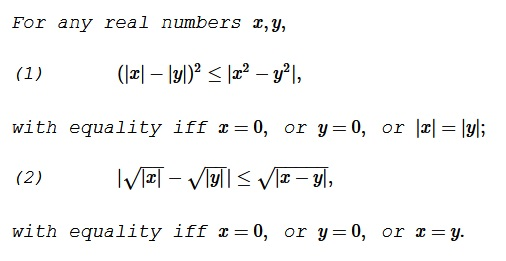# Simple Yet Uncommon Inequalities with Absolute Value

### Problem### Solution of (1)

For convenience, replace (1) with $(|x|-|y|)^2\le ||x|^2-|y|^2|.\,$ WLOG, assume $|x|\ge |y|.\,$ Then the inequality reduces to

$|x|^2-2\cdot |x|\cdot |y|+|y|^2\le |x|^2-|y|^2,$

or, $2\cdot |y|^2\le 2\cdot |x|\cdot |y|,\,$ which is true by our assumption.

There is clearly equality when $|x|=|y|\,$ or when one of $x,y\,$ equals $0.$

### Solution of (2)

Assume first $x\ge y\ge 0.\,$ Then we have to prove that \sqrt{x}-\sqrt{y}\le \sqrt{x-y}.\,$By squaring we get an inequality reminiscent of (1):$x+y-2\sqrt{xy}\le x-y,$or,$2y\le 2\sqrt{xy}\,$which is true, due to our assumption. Equality is attained when$x=y,\,$or either$x=0\,$or$y=0.$Assume not that$x\ge 0\ge y\,$and let$z=-y.\,$After squaring the inequality becomes$x+z-2\sqrt{xz}\le x+z,$which is obvious. With equality when$x=0\,$or$y=0.\,$Owning to the symmetry of the absolute value$(|-a|=|a|)\,\$ there's no need to consider additional cases.

### Acknowledgment

This is theorem 11 from D. S. Mitrinović's Analytic Inequalities (This is an expensive book but is also available as a pdf from several sites.)

Inequalities in Two Variables